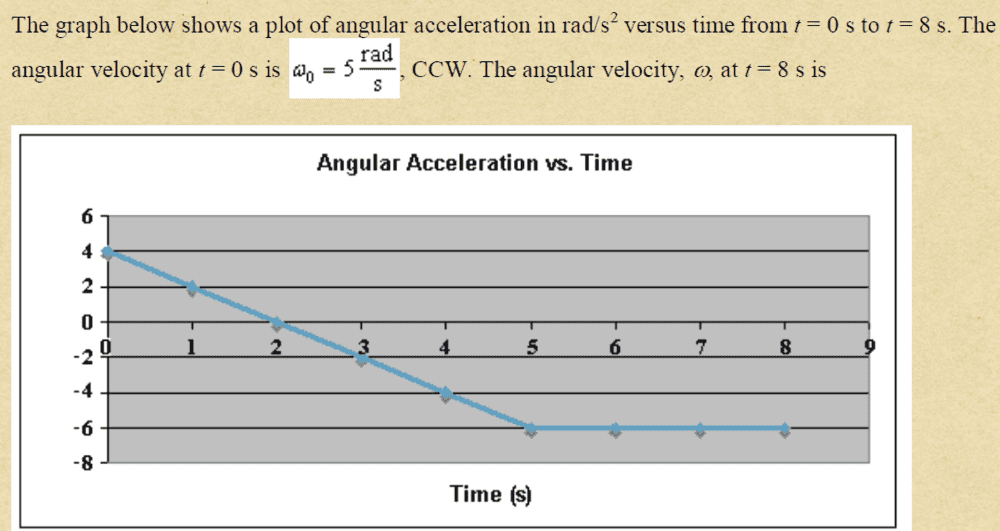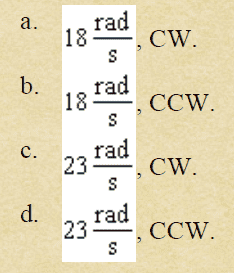# Getting the angular velocity using the angular acceleration graph

• dahoom102

#### dahoom102

Homework Statement
The graph below shows a plot of angular acceleration in rad/s2 from t=0 to t = 8s, the angular velocity at t=0 is 5rad/s ccw. the angular velocity at t=8 is
Relevant Equations
α=dω/dθWhat i did is getting the area as follows,
2×4×1/2 +3×-6×1/2 +4×-6 = -29
and then use this
Δω=ωf-ωi
-29=ωf-5
ωf=24
but there is no such choice.

##\alpha = \dfrac{d\omega}{dt}=\omega \dfrac{d\omega}{d \theta} .~~##•